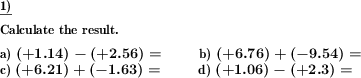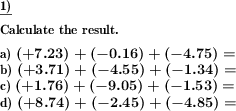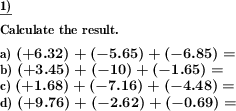Custom math worksheets at your fingertips# Details for problem "Negative decimals add and subtract"

Quickname: 2601

Elementary School, Primary School, Junior High School, Middle School, High School.

## Summary

Negative decimal numbers are to be added and subtracted

## Examples## Description

Calculate with negative decimal numbers and decimal fractions. The problems are presented either as addition or subtraction problems or mixed problems.

The number of problems, the number space and the number of decimal places can be selected.

To simplify the tasks slightly, it can be specified that the first summand will always be positive.

Download free printable worksheets for this math problem here. The worksheet contains the problems only, the solution sheet includes the answers. Just click on the respective link.

•Worksheet 1Solution sheet with answers
•Worksheet 2Solution sheet with answers
•Worksheet 3Solution sheet with answers

If you can not see the solution sheets for download, they may be filtered out by an ad blocker that you may have installed. If this is the case, please allow ads for this page and reload the page. The solution sheets will then reappear.

• Do these sample worksheets do not really fit?
• Do you need more math worksheets, with a different level of difficulty?
• Would you like to combine different problems on a worksheet and adjust them to your needs?
• As a teacher, you can put together your own worksheets using the automatically generated math problems provided.
With a free initial credit, you can start creating your own math worksheets in a few minutes.

You can try it for free! Register here, to create custom worksheets now!

## Customization options for this problem

Parameter
Possible values
Number of problems
1, 2, 3, 4, 5, 6, 7, 8, 9, 10
Number range
1, 10, 20, 50, 100
Mixed, Plus, Minus
Number of summands
2, 3, 4
First summand negative
never, can be
Number of decimal places
1, 2, 3, 4, 5, 6

## Similar problems

Remark
Description
With whole numbers
Negative whole numbers are to be added and subtracted